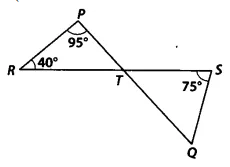Q

# In Fig. 6.42, if lines PQ and RS intersect at point T, such that ∠ PRT = 40°, ∠ RPT = 95° and ∠ TSQ = 75°, find ∠ SQT.

4. In Fig. 6.42, if lines PQ and RS intersect at point T, such that PRT = 40°, RPT = 95° and TSQ = 75°, find SQT.ViewsWe have,
lines PQ and RS intersect at point T, such that PRT = 40°, RPT = 95° and TSQ = 75°

In PRT, by using angle sum property
PRT + PTR + TPR =
So, PTR  =

Since lines, PQ and RS intersect at point T
therefore, PTR = QTS (Vertically opposite angles)
QTS =

Now, in QTS,
By using angle sum property
TSQ + STQ + SQT =
So, SQT =

Exams
Articles
Questions